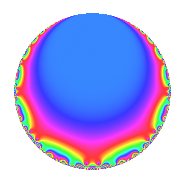# Properties

 Label 1200.2.a.eLevel 1200 Weight 2 Character orbit 1200.a Self dual Yes Analytic conductor 9.582 Analytic rank 0 Dimension 1 CM No Inner twists 1

# Learn more about

## Newspace parameters

 Level: $$N$$ = $$1200 = 2^{4} \cdot 3 \cdot 5^{2}$$ Weight: $$k$$ = $$2$$ Character orbit: $$[\chi]$$ = 1200.a (trivial)

## Newform invariants

 Self dual: Yes Analytic conductor: $$9.58204824255$$ Analytic rank: $$0$$ Dimension: $$1$$ Coefficient field: $$\mathbb{Q}$$ Coefficient ring: $$\mathbb{Z}$$ Coefficient ring index: $$1$$ Fricke sign: $$-1$$ Sato-Tate group: $\mathrm{SU}(2)$

## $q$-expansion

 $$f(q)$$ $$=$$ $$q$$ $$\mathstrut -\mathstrut q^{3}$$ $$\mathstrut +\mathstrut q^{9}$$ $$\mathstrut +\mathstrut O(q^{10})$$ $$q$$ $$\mathstrut -\mathstrut q^{3}$$ $$\mathstrut +\mathstrut q^{9}$$ $$\mathstrut +\mathstrut 4q^{11}$$ $$\mathstrut +\mathstrut 2q^{13}$$ $$\mathstrut -\mathstrut 2q^{17}$$ $$\mathstrut -\mathstrut 4q^{19}$$ $$\mathstrut -\mathstrut q^{27}$$ $$\mathstrut -\mathstrut 2q^{29}$$ $$\mathstrut -\mathstrut 4q^{33}$$ $$\mathstrut +\mathstrut 10q^{37}$$ $$\mathstrut -\mathstrut 2q^{39}$$ $$\mathstrut +\mathstrut 10q^{41}$$ $$\mathstrut +\mathstrut 4q^{43}$$ $$\mathstrut +\mathstrut 8q^{47}$$ $$\mathstrut -\mathstrut 7q^{49}$$ $$\mathstrut +\mathstrut 2q^{51}$$ $$\mathstrut +\mathstrut 10q^{53}$$ $$\mathstrut +\mathstrut 4q^{57}$$ $$\mathstrut +\mathstrut 4q^{59}$$ $$\mathstrut -\mathstrut 2q^{61}$$ $$\mathstrut +\mathstrut 12q^{67}$$ $$\mathstrut +\mathstrut 8q^{71}$$ $$\mathstrut -\mathstrut 10q^{73}$$ $$\mathstrut +\mathstrut q^{81}$$ $$\mathstrut +\mathstrut 12q^{83}$$ $$\mathstrut +\mathstrut 2q^{87}$$ $$\mathstrut -\mathstrut 6q^{89}$$ $$\mathstrut -\mathstrut 2q^{97}$$ $$\mathstrut +\mathstrut 4q^{99}$$ $$\mathstrut +\mathstrut O(q^{100})$$

## Embeddings

For each embedding $$\iota_m$$ of the coefficient field, the values $$\iota_m(a_n)$$ are shown below.

For more information on an embedded modular form you can click on its label.

Label $$\iota_m(\nu)$$ $$a_{2}$$ $$a_{3}$$ $$a_{4}$$ $$a_{5}$$ $$a_{6}$$ $$a_{7}$$ $$a_{8}$$ $$a_{9}$$ $$a_{10}$$
1.1
 0
0 −1.00000 0 0 0 0 0 1.00000 0
 $$n$$: e.g. 2-40 or 990-1000 Significant digits: Format: Complex embeddings Normalized embeddings Satake parameters Satake angles

## Inner twists

This newform does not admit any (nontrivial) inner twists.

## Atkin-Lehner signs

$$p$$ Sign
$$2$$ $$-1$$
$$3$$ $$1$$
$$5$$ $$1$$

## Hecke kernels

This newform can be constructed as the intersection of the kernels of the following linear operators acting on $$S_{2}^{\mathrm{new}}(\Gamma_0(1200))$$:

 $$T_{7}$$ $$T_{11}$$ $$\mathstrut -\mathstrut 4$$ $$T_{13}$$ $$\mathstrut -\mathstrut 2$$